Creating, Formatting Excel with various options using ByteScout Spreadsheet SDK in C# - Part 1 - ByteScout
• Home
• /
• Blog
• /
• Creating, Formatting Excel with various options using ByteScout Spreadsheet SDK in C# – Part 1

# Creating, Formatting Excel with various options using ByteScout Spreadsheet SDK in C# – Part 1

Microsoft Excel is used in almost all types of industries and professions. Bankers, accountants, consultants, analysts, etc. all use an excel on a consistent basis. As a developer, you will often be given a requirement to programmatically generate the interactive excel worksheet either for reporting purposes or for presentation purposes. ByteScout Spreadsheet SDK has provided, in no particular order, and out of box features to formatting and manipulating the excel file.

In this article, we are going to explore the various options available to format the excel by writing a step-by-step program to create Excel C#. It lets developers create rich Excel file from the formatting perspective.

### Take a look at the main highlights in this tutorial:

Let’s get started:

## * Create an Excel file with merged cells in C#:

Let’s write this step-by-step.

Step-1: Create a new C# console application project.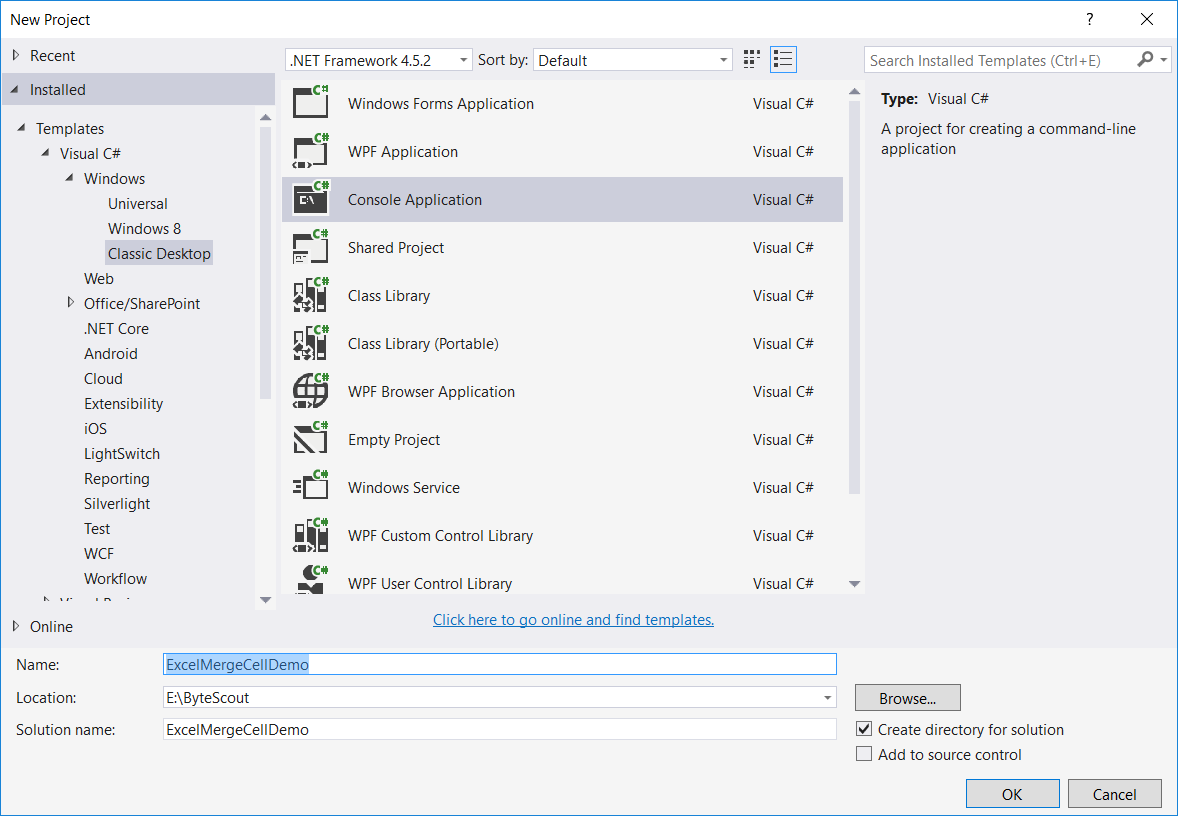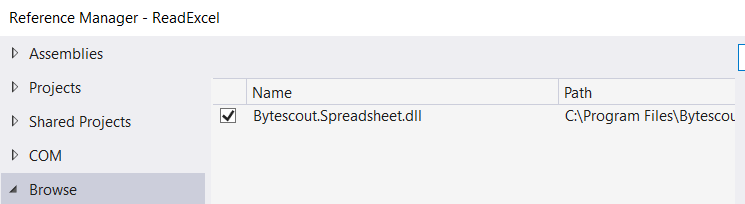Step-3: Include the following namespaces in the Program.cs file.

`using System.Diagnostics;`
`using System.Drawing;`
`using System.IO;`
`using Bytescout.Spreadsheet;`
`using Bytescout.Spreadsheet.Constants;`

Step-4: Add the following code snippet to merge cells in an Excel file.

```class Program
{
static void Main(string[] args)
{
{
// Make two column
worksheet.Cell(0, 0).Value = "Product Name";
worksheet.Cell(0, 1).Value = "Product Type";
// Set font bold for headers
worksheet.Cell(0, 0).Font = new Font("Verdana", 12, FontStyle.Bold);
worksheet.Cell(0, 1).Font = new Font("Verdana", 12, FontStyle.Bold);

// Write product name
worksheet.Cell(1, 0).Value = "Refrigerator";
worksheet.Cell(2, 0).Value = "TV";
worksheet.Cell(3, 0).Value = "Washing Machine";
worksheet.Cell(4, 0).Value = "AC";
worksheet.Cell(5, 0).Value = "Mobile";

//Merge cells
worksheet.Range("B2:B6").Merge();
//Write text
worksheet.Cell("B6").MergedWithCell.Value = "Electronic Goods";
worksheet.Cell("B6").MergedWithCell.AlignmentVertical = AlignmentVertical.Centered;

// Set the column to be autofit as per the content.
worksheet.Columns.AutoFit();
worksheet.Columns.AutoFit();

if (File.Exists("MergedCellsDemo.xls"))
File.Delete("MergedCellsDemo.xls");

// Save document
document.SaveAs("MergedCellsDemo.xls");

document.Close();

// Open generated XLS document
Process.Start("MergedCellsDemo.xls");
}
}
}
```

And the final excel file would look something like this –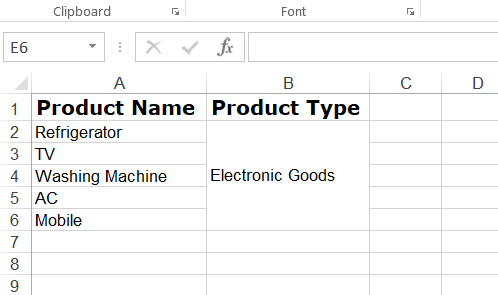Here’s the demo output.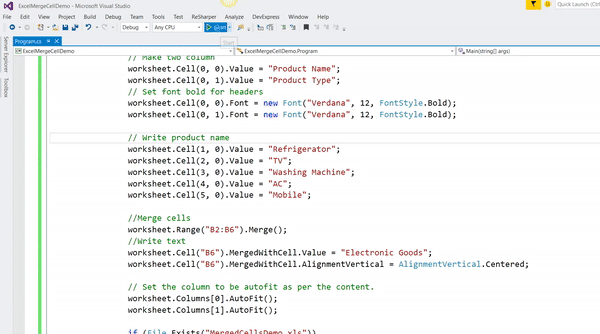Let’s check the above code step by step.

• Merging means simply combining multiple cells. So, first, we create an instance of a Spreadsheet object.

`// Create new Spreadsheet`

`Spreadsheet document = new Spreadsheet();`

• Add the new worksheet in the newly created object of Spreadsheet using the following code.

`// add a new worksheet`

`Worksheet Sheet = document.Workbook.Worksheets.Add("Sheet1");   `

• Set two columns – “`Product Name`” and “`Product Type`“, set its font and font color and then write some sample text.

`// Make two column`

`worksheet.Cell(0, 0).Value = "Product Name";`

`worksheet.Cell(0, 1).Value = "Product Type";`

`// Set font bold for headers`

`worksheet.Cell(0, 0).Font = new Font("Verdana", 12, FontStyle.Bold);`

`worksheet.Cell(0, 1).Font = new Font("Verdana", 12, FontStyle.Bold);`

`// Write product name`

`worksheet.Cell(1, 0).Value = "Refrigerator";`

`worksheet.Cell(2, 0).Value = "TV";`

`worksheet.Cell(3, 0).Value = "Washing Machine";`

`worksheet.Cell(4, 0).Value = "AC";`

`worksheet.Cell(5, 0).Value = "Mobile";`

• Merge the cells. column header “Product Type” is at “B1” position. So, merging of cells will start from “B2” to “B6” as we enter 5 products in the file.

`//Merge cells`

`worksheet.Range("B2:B6").Merge();`

• Write the text in merged cells and align its text as centered.

`// Write text in merged cell`

`worksheet.Cell("B6").MergedWithCell.Value = "Electronic Goods";`

`worksheet.Cell("B6").MergedWithCell.AlignmentVertical = AlignmentVertical.Centered;`

• Set the width of a column to be automatically fit as per the column content.

`// Set the column to be autofit as per the content.`

`worksheet.Columns.AutoFit();`

`worksheet.Columns.AutoFit();`

• Check if the same file name already exists in the application’s bin\Debug folder. If exists then first delete it.

`// delete MyNewExcelFile file if exists already`

`if (File.Exists("MergedCellsDemo.xls")){`

`   File.Delete("MergedCellsDemo.xls");`

`}`

• Generate output file

`// Save document`

`document.SaveAs("MergedCellsDemo.xls");`

## * Create an Excel file with page break (horizontal and vertical) in a worksheet:

This feature of Excel is reasonably useful when there is a large number of data in excel and you want to print it. So when you print, your content on the sheet will automatically be divided into the pages. But you know what you can control this automatic page breaks. Yes, you can set your own page breaks programmatically. You can easily achieve this requirement using ByteScout Spreadsheet.

In this program, we will insert horizontal and vertical page breaks in a worksheet.

Step-1: Create a new C# console application project as shown in the first demo program.

Step-3: Include the following namespaces in the Program.cs file.

`using System.IO;`

`using System.Diagnostics;`

`using System.Drawing;`

`using Bytescout.Spreadsheet;`

`using Bytescout.Spreadsheet.Structures;`

Step-4: Add the following code snippet.

```class Program
{
static void Main(string[] args)
{
{

// Make two column
worksheet.Cell(0, 0).Value = "Product Name";
worksheet.Cell(0, 1).Value = "Product Type";

// Set font bold for headers
worksheet.Cell(0, 0).Font = new Font("Verdana", 12, FontStyle.Bold);
worksheet.Cell(0, 1).Font = new Font("Verdana", 12, FontStyle.Bold);

// Write product name
worksheet.Cell(1, 0).Value = "Refrigerator";
worksheet.Cell(1, 1).Value = "Electronic Goods";

// Save document
document.Close();

// Open generated XLS document
}
}
}
```

When you run this program, it opens Excel file in Normal view. But when you click on “Page Layout” at the bottom-right side of excel as shown in the following image.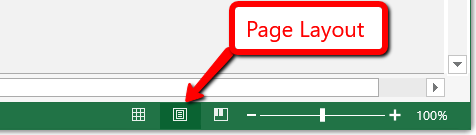You will be able to see our own page breaks that we set programmatically using the following code.

`// Add horizontal page break`

`worksheet.HPageBreaks.Add(new CellsRange("A11"));`

`// Add vertical page break`

`worksheet.VPageBreaks.Add(new CellsRange("G1"));`

And it would look something like this: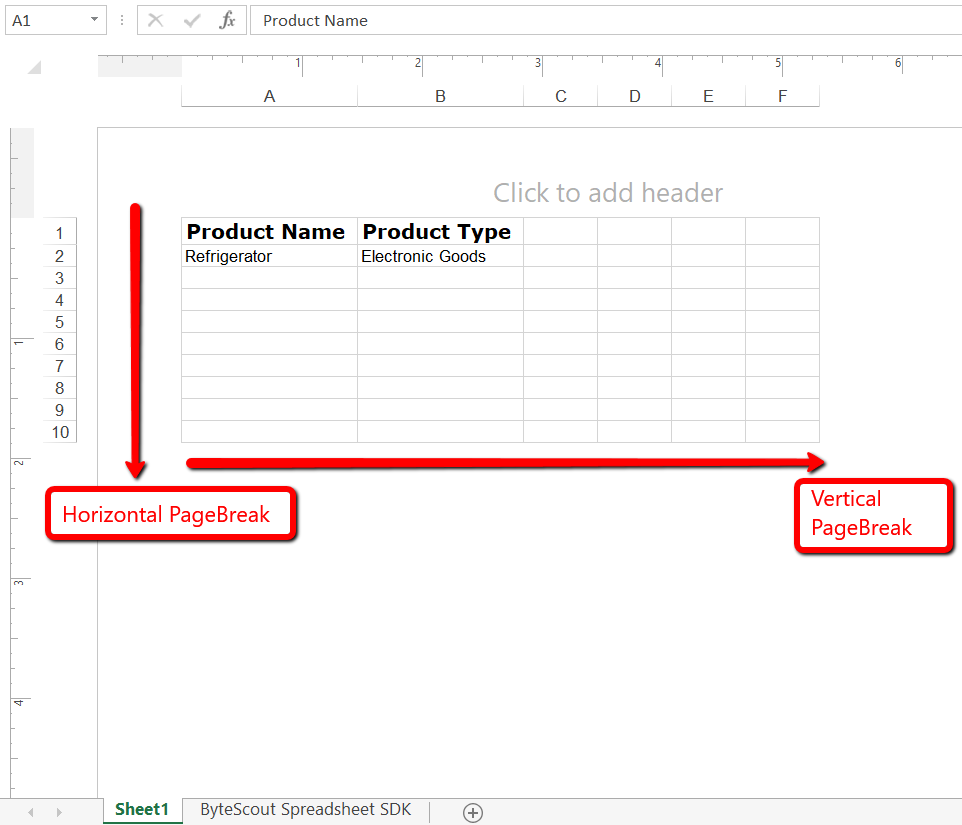Here’s the demo output: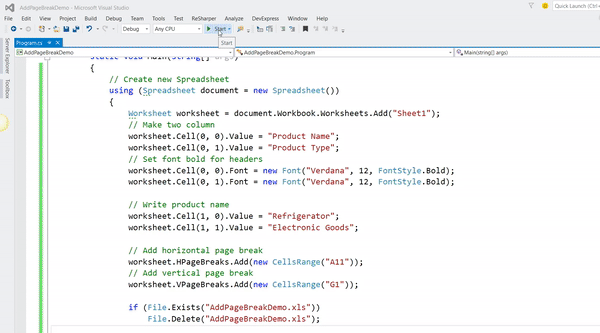## * Create a formatted Excel worksheet in C#:

You will often have to give a requirement to programmatically generate the formatted excel worksheet for some sales projections or for any kind of reporting purpose. Fortunately, making a formatted excel worksheet is much simple with ByteScout Spreadsheet SDK.

In this program, we will see how to create an Excel file that would look like this: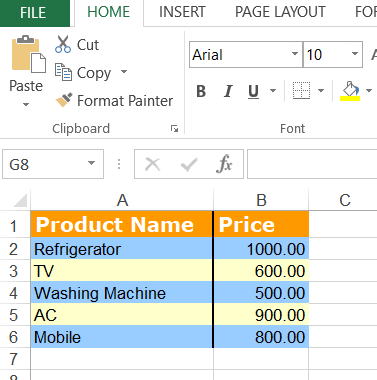Step-1: Create a new C# console application project.

Step-3: Include the following namespaces in the Program.cs file.

`using System.Diagnostics;`
`using System.IO;`
`using System.Drawing;`
`using Bytescout.Spreadsheet;`
`using Bytescout.Spreadsheet.Constants;`

Step-4: Add the following code snippet.

```class Program
{
static void Main(string[] args)
{
{
// Make two column
worksheet.Cell(0, 0).Value = "Product Name";
worksheet.Cell(0, 1).Value = "Price";

worksheet.Cell(0, 0).Font = new Font("Verdana", 12, FontStyle.Bold);
worksheet.Cell(0, 0).FontColor = Color.White;
worksheet.Cell(0, 0).RightBorderStyle = LineStyle.Medium;
worksheet.Cell(0, 0).FillPattern = PatternStyle.Solid;
worksheet.Cell(0, 0).FillPatternForeColor = Color.DarkOrange;  // Also use - Color.FromArgb(11, 255, 255);

worksheet.Cell(0, 1).Font = new Font("Verdana", 12, FontStyle.Bold);
worksheet.Cell(0, 1).FontColor = Color.White;
worksheet.Cell(0, 1).FillPattern = PatternStyle.Solid;
worksheet.Cell(0, 1).FillPatternForeColor = Color.DarkOrange;   // Also use - Color.FromArgb(11, 255, 255);

// Write product name
worksheet.Cell(1, 0).Value = "Refrigerator";
worksheet.Cell(2, 0).Value = "TV";
worksheet.Cell(3, 0).Value = "Washing Machine";
worksheet.Cell(4, 0).Value = "AC";
worksheet.Cell(5, 0).Value = "Mobile";
// Write product price
worksheet.Cell(1, 1).Value = 1000;
worksheet.Cell(2, 1).Value = 600;
worksheet.Cell(3, 1).Value = 500;
worksheet.Cell(4, 1).Value = 900;
worksheet.Cell(5, 1).Value = 800;

for (int i = 1; i &lt;= 5; i++)
{
worksheet.Cell(i, 0).FillPattern = PatternStyle.Solid;
worksheet.Cell(i, 0).RightBorderStyle = LineStyle.Medium;
worksheet.Cell(i, 1).FillPattern = PatternStyle.Solid;
worksheet.Cell(i, 1).NumberFormatString = "0.00";
if (i % 2 == 0)
{
worksheet.Cell(i, 0).FillPatternForeColor = Color.LightYellow;
worksheet.Cell(i, 1).FillPatternForeColor = Color.LightYellow;
}
else
{
worksheet.Cell(i, 0).FillPatternForeColor = Color.LightBlue;
worksheet.Cell(i, 1).FillPatternForeColor = Color.LightBlue;
}
}

worksheet.Columns.AutoFit();
worksheet.Columns.AutoFit();

if (File.Exists("InteractiveExcelDemo.xls"))
File.Delete("InteractiveExcelDemo.xls");

//Save document
document.SaveAs("InteractiveExcelDemo.xls");
document.Close();

// Open generated XLS document
Process.Start("InteractiveExcelDemo.xls");
}
}
}
```

Here’s the output: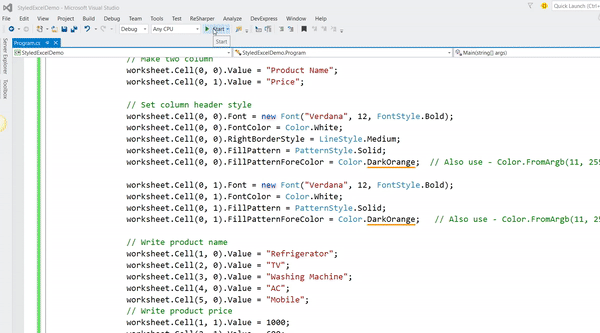Let’s try to understand the above code.

• In the above code, we simply create an Excel which contains data in tabular format with two column – “Product Name” and “Price”. So first we set the header style. We set the header text to bold with orange background and white font color.

`// Set column header style`
`worksheet.Cell(0, 0).Font = new Font("Verdana", 12, FontStyle.Bold);`
`worksheet.Cell(0, 0).FontColor = Color.White;`
`worksheet.Cell(0, 0).RightBorderStyle = LineStyle.Medium;`
`worksheet.Cell(0, 0).FillPattern = PatternStyle.Solid;`
`worksheet.Cell(0, 0).FillPatternForeColor = Color.DarkOrange;  // Also use - Color.FromArgb(11, 255, 255);`

• And then in the table row data, we set the alternate background color to light yellow and light blue. Here we also format our price column using the following code.

`for (int i = 1; i <= 5; i++)`
`{`
`    worksheet.Cell(i, 0).FillPattern = PatternStyle.Solid;`
`    worksheet.Cell(i, 0).RightBorderStyle = LineStyle.Medium;`
`    worksheet.Cell(i, 1).FillPattern = PatternStyle.Solid;`
`    worksheet.Cell(i, 1).NumberFormatString = "0.00";`
`    if (i % 2 == 0)`
`    {`
`        worksheet.Cell(i, 0).FillPatternForeColor = Color.LightYellow;`
`        worksheet.Cell(i, 1).FillPatternForeColor = Color.LightYellow;`
`    }`
`    else`
`    {`
`        worksheet.Cell(i, 0).FillPatternForeColor = Color.LightBlue;`
`        worksheet.Cell(i, 1).FillPatternForeColor = Color.LightBlue;`
`    }`
`}`

## * Create excel worksheet with hidden rows/columns in C#

Assume that you would have given an excel file with so many columns for analysis. In that type of situation, it would be really helpful to hide some unwanted columns or rows. In this program, we will be going to see how to create an excel file with some hidden rows/columns.

Step-1: Create a new C# console application project.

Step-3: Include the following namespaces in the Program.cs file.

`using System.Diagnostics;`
`using System.IO;`
`using Bytescout.Spreadsheet`

Step-4: Add the following code snippet.

```class Program
{
static void Main(string[] args)
{
{

// Hide first two columns
worksheet.Columns.Hidden = true;
worksheet.Columns.Hidden = true;

// Hide 4th and 5th no# of rows
worksheet.Rows.Hidden = true;
worksheet.Rows.Hidden = true;

if (File.Exists("HideRowColumnDemo.xls"))
File.Delete("HideRowColumnDemo.xls");
//Save document
document.SaveAs("HideRowColumnDemo.xls");

document.Close();

// Open generated XLS document
Process.Start("HideRowColumnDemo.xls");
}
}
}
```

If you run the application, the final Excel file will look like this: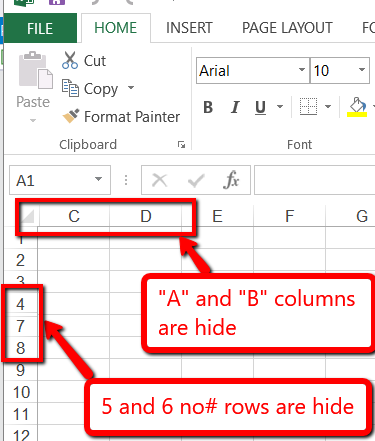As you can see in the above code snippet which itself is self-explanatory where we hide column “A” and “B” and then hide row no# 4 and 5 using the following code.

`// Hide first two columns`
`worksheet.Columns.Hidden = true;`
`worksheet.Columns.Hidden = true;`

`// Hide 4th and 5th no# of rows`
`worksheet.Rows.Hidden = true;`
`worksheet.Rows.Hidden = true;`

## * Add / manipulate images in excel worksheet in C#

In this program, we will see how to add/manipulate images in an excel file. Explanation purpose, we have used ByteScout logo in the worksheet and will see the different options to manipulate it.

Step-1: Create a new C# console application project.

Step-3: Include the following namespaces in the Program.cs file.

`using System.Diagnostics;`
`using System.IO;`
`using System.Drawing;`
`using Bytescout.Spreadsheet;`
`using Bytescout.Spreadsheet.MSODrawing;`

Step-4: Add the following code snippet.

```class Program
{
static void Main(string[] args)
{
{

// Put an image to "C3" cell
PictureShape shape = worksheet.Pictures.Add(2, 2, "ByteScout-Logo.png");

// Make the picture "floating". It will be not moved if you move or resize the "C3" cell
shape.PlacementType = Placement.FreeFloating;

// Make the picture brighter
shape.Brightness = 0.68f;

// Put second image to "K11" cell

// Make the picture bound to the cell. It will be moved along with the "K11" cell
shape.PlacementType = Placement.MoveAndSize;

// Crop 10% from left and right side of the image
shape.CropFromLeft = 0.05f;
shape.CropFromRight = 0.05f;

if (File.Exists("WithImageDemo.xls"))
File.Delete("WithImageDemo.xls");

// Save document
document.SaveAs("WithImageDemo.xls");

document.Close();

// Open generated XLS document
Process.Start("WithImageDemo.xls");
}
}
}
```

If you run the application, the final Excel file will look like this: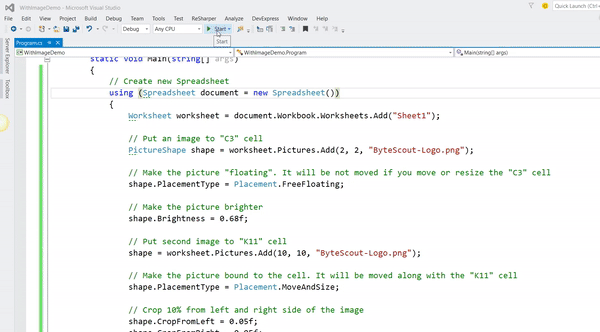Let’s try to understand the above code.

• In the above code, we have added “ByteScout logo” at two locations. First, put an image to “C3” cell using the following code.

`// Put an image to "C3" cell`
`PictureShape shape = worksheet.Pictures.Add(2, 2, "ByteScout-Logo.png");`

• Set the picture brightness and make the picture “floating” by setting the PlacementType property. It means that the image will not be moved if you move or resize the “C3” cell.

`// Make the picture brighter`
`shape.Brightness = 0.68f;`

`// It will be not moved if you move or resize the "C3" cell`
`shape.PlacementType = Placement.FreeFloating;`

• Put an image to “K11” cell using the following code.

`// Put second image to "K11" cell`
`shape = worksheet.Pictures.Add(10, 10, "ByteScout-Logo.png");`

• In this case, we make the picture “MoveAndSize” by setting the PlacementType property. It means that image will be moved along with the “K11” cell. Here we also crop the image from the left and right.

`// Make the picture bound to the cell. It will be moved along with the "K11" cell`
`shape.PlacementType = Placement.MoveAndSize;`

`// Crop 10% from left and right side of the image`
`shape.CropFromLeft = 0.05f;`
`shape.CropFromRight = 0.05f;`

## * Delete rows/columns from excel worksheet in C#

In this program, we will be going to see that how can programmatically remove the rows/columns from the existing file. As shown in the below image, assume that you want to remove the “Price” column and last two rows from the file.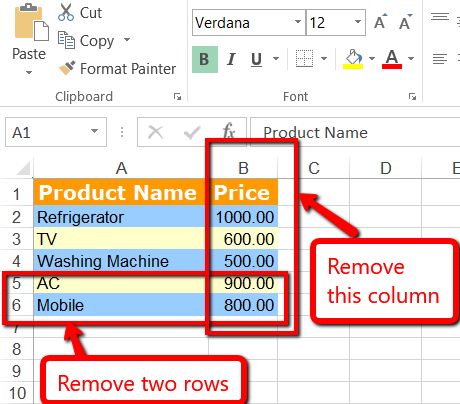The final Excel file would look something like this: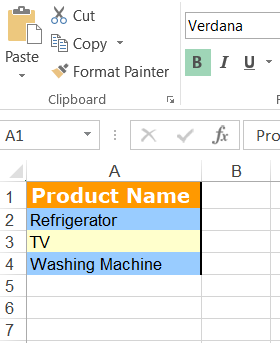Step-1: Create a new C# console application project.

Step-3: Include the following namespaces in the Program.cs file.

`using System.Diagnostics;`
`using System.IO;`
`using Bytescout.Spreadsheet;`

Step-4: Add the following code snippet.

```class Program
{
static void Main(string[] args)
{
{

// Get Worksheet
Worksheet worksheet = document.Workbook.Worksheets;

// Remove row from the above mentioned excel file
worksheet.Rows.Delete(4, 5);
// Remove 2nd column from the above mentioned excel file
worksheet.Columns.Delete(1);

if (File.Exists("DeleteRowColumnsDemo.xls"))
File.Delete("DeleteRowColumnsDemo.xls");

// Save document
document.SaveAs("DeleteRowColumnsDemo.xls");

document.Close();

// Open generated XLS document
Process.Start("DeleteRowColumnsDemo.xls");
}
}
}
```

If you run the application, the final Excel file will look like this: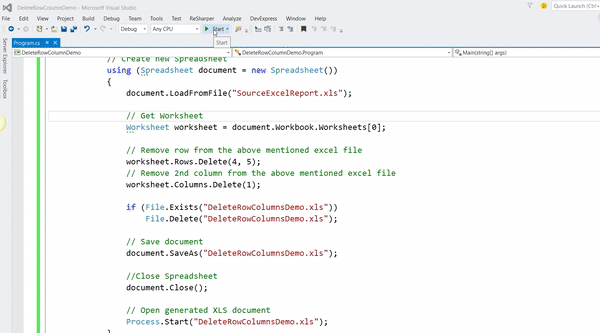In this part-1 article, we’ve explored different features for formatting and manipulating the Excel. ByteScout Spreadsheet SDK has many more features and we will explore some more features in the next article.ByteScout Team of Writers ByteScout has a team of professional writers proficient in different technical topics. We select the best writers to cover interesting and trending topics for our readers. We love developers and we hope our articles help you learn about programming and programmers.